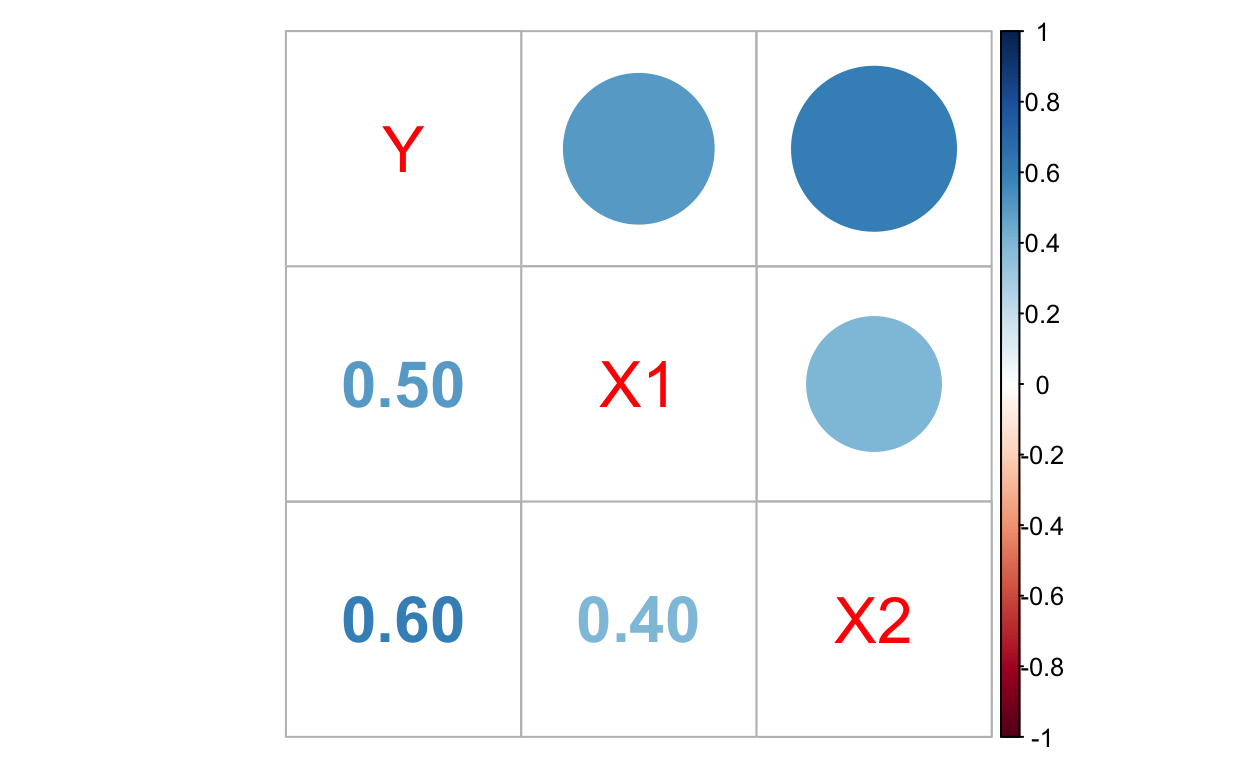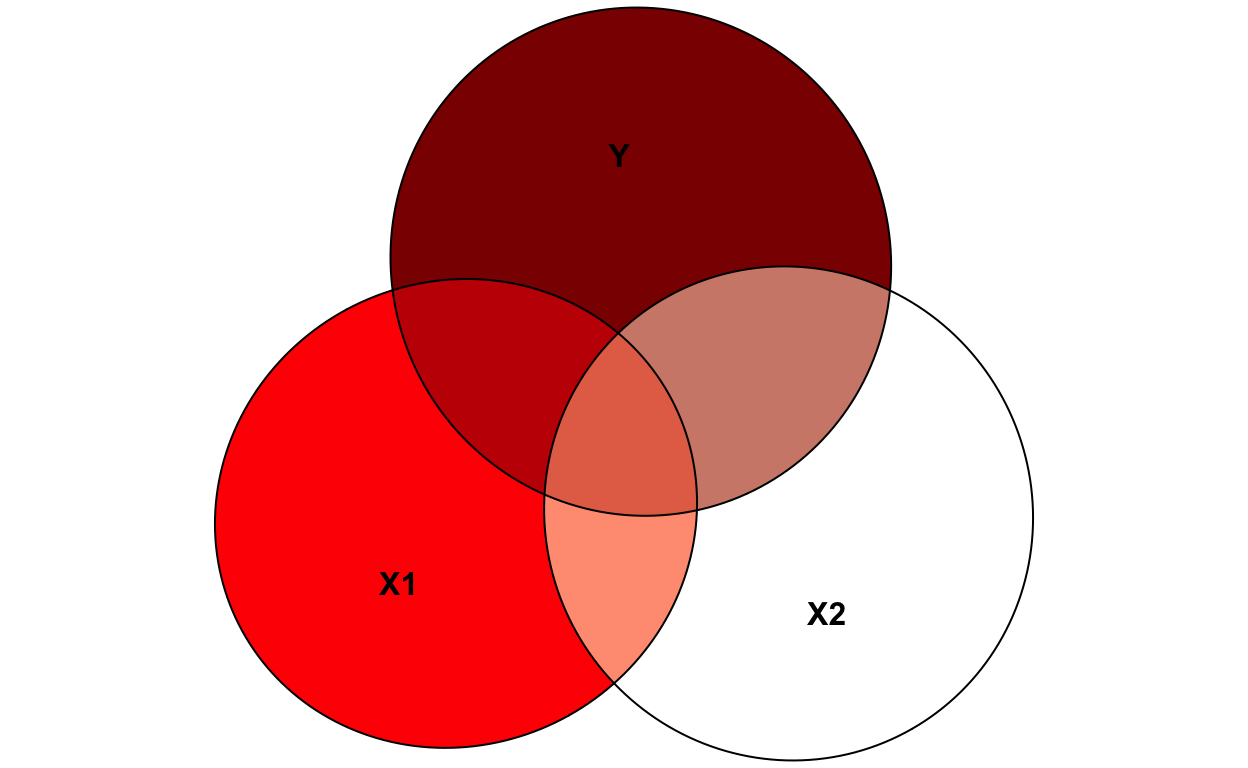# Residualization reinterpretation

Nicholas Judd https://staff.ki.se/people/nicholas-judd (Karolinska Institute)https://ki.se/en , Dr. Bruno Sauce https://brunosauce.net/ (Vrije University)https://research.vu.nl/en/persons/bruno-sauce-silva

## How standardized effects vary with residualization

The Frisch-Waugh-Lovell theorem theorem states that residualizing all variables in the linear model for a variable (e.g., X2) is equal to adding it as a covariate.

Therefore B1 in the two equations are identical:

$\operatorname{Y} = \alpha + \beta_{1}(\operatorname{X1}) + \beta_{2}(\operatorname{X2}) + \epsilon$ $\operatorname{Y_{residualized\_X2}} = \alpha + \beta_{1}(\operatorname{X1_{residualized\_X2}}) + \epsilon$

This is a quick simulation showing the Frisch-Waugh-Lovell with two correlated predictors. It also shows how residualizing only the dependent variable leads to a different result and how rescaling can artificially inflate your standardized effect.

### Data simulation

First we simulate data with 1000 subjects:• 3 correlated variables in standard units
• Y reflects the dependent variable while X1 & X2 are predictors
• We have X2 as a predictor in two linear models with Y & X1 as DVs to extract the residuals
• These new variables are coded as Y_X2res & X1_X2resTable 1: An overview of simluated variables
n mean sd
Y 1000 0 1.00
X1 1000 0 1.00
X2 1000 0 1.00
Y_X2res 1000 0 0.80
X1_X2res 1000 0 0.92

### 1. Frisch-Waugh-Lovell replication

In the table below we can see that the effect size and the confidence intervals of X1 are the same when we residualize both the dependent and independent variable.

Y Y_X2res
Predictors Estimates CI p Estimates CI p
(Intercept) -0.00 -0.05 – 0.05 1.000 -0.00 -0.05 – 0.05 1.000
X1 0.31 0.26 – 0.36 <0.001
X2 0.48 0.43 – 0.53 <0.001
X1_X2res 0.31 0.26 – 0.36 <0.001

### 2. Scaling inflates effect sizes

$\dfrac{\beta_{1}*\operatorname{SD_{X}}}{SD_{Y}}$

If we standardize our residualized model it will inflate (mess wtih) the effect sizes. This is because we are rescaling it (see equation above). While this may seem obvious at first it can sneak up on you, for example if you fit a structural equation model where you residualized the variable for age and now you standardize it. As you can see on the equation above it will either inflate or deflate depending on the amount of signal X2 accounts for in Y or X1.

Y Y_X2res
Predictors std. Beta std. Beta
(Intercept) 0.00 -0.00
X1 0.31
X2 0.48
X1_X2res 0.35

### 3. Residualizing only the DV changes our interpretation

When we only residualize the dependent variable it changes the meaning of the other term when the two are related. This is because the common variance between X1 & X2 is being thrown out.

Y Y_X2res
Predictors Estimates std. Beta Estimates std. Beta
(Intercept) -0.00 0.00 -0.00 -0.00
X1 0.31 0.31 0.26 0.32
X2 0.48 0.48

### 4. Residualizing only the IV changes our confidence

This one is problematic, as the magnitude of the effect stays the same yet the SE, and in turn, the p-vals differ!

Y Y Y_X2res
Predictors Estimates std. Error Estimates std. Error Estimates std. Error
(Intercept) -0.000 0.024 -0.000 0.030 -0.000 0.024
X1 0.310 0.026
X2 0.476 0.026
X1_X2res 0.310 0.033 0.310 0.026

If we accidentally scale X1_X2res in the model with Y as the predictor, it will default our effect size since Y continues to have a sd of 1 while X1_X2res is now less.

To drive the point home of different standard errors, here are the models refit to the first 80 subjects…

Y Y Y_X2res
Predictors Estimates std. Error p Estimates std. Error p Estimates std. Error p
(Intercept) 0.041 0.096 0.672 0.122 0.118 0.304 -0.000 0.094 1.000
X1 0.248 0.113 0.031
X2 0.603 0.108 <0.001
X1_X2res 0.248 0.139 0.079 0.248 0.112 0.030

### Bottom line

Try to be careful because it can easily change the nature of the actual effect, the size of the effects (e.g., scaling), and our confidence of the effect (i.e., p-vals/SE). :)